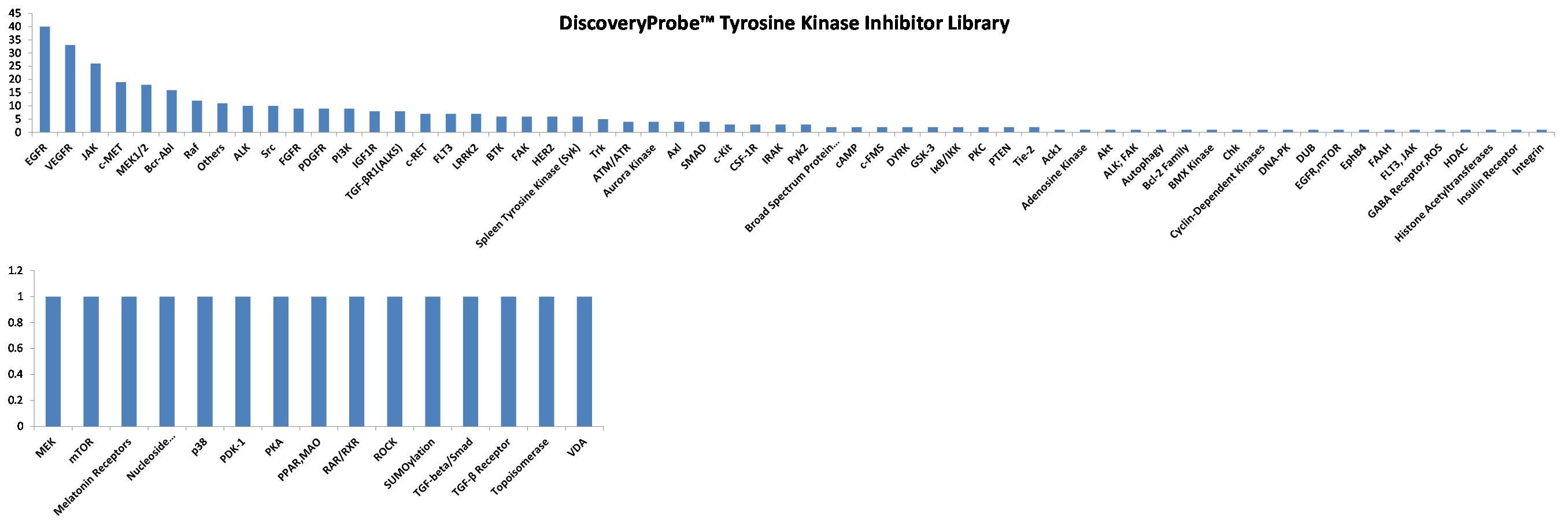Close
• Setting

# DiscoveryProbe™ Tyrosine Kinase Inhibitor Library

Catalog No.
L1028
A unique collection of 369 tyrosine kinase inhibitors for high throughput screening (HTS) and high content screening (HCS).Grouped product items
SizePriceStock Qty
100uL/well(10 mM solution)
\$5,770.00
In stock
250uL/well(10 mM solution)
\$10,290.00
In stock

Tel: +1-832-696-8203

Email: [email protected]

Worldwide Distributors

## Featured Products of the Library

 Catalog No. Product Name Summary Targets CAS Number Smiles A1389 WZ4002 Mutant-selective EGFR inhibitor(L858R,T790M), irreversible and potent EGFR 1213269-23-8 CN1CCN(CC1)C2=CC(=C(C=C2)NC3=NC=C(C(=N3)OC4=CC=CC(=C4)NC(=O)C=C)Cl)OC A2838 OSI-930 Inhibitor of Kit, KDR, Flt, CSF-1R, c-Raf and Lck c-Kit 728033-96-3 C1=CC=C2C(=C1)C(=CC=N2)CNC3=C(SC=C3)C(=O)NC4=CC=C(C=C4)OC(F)(F)F A3014 BGJ398 FGFR inhibitor ,potent and selective FGFR 872511-34-7 CCN1CCN(CC1)C2=CC=C(C=C2)NC3=CC(=NC=N3)N(C)C(=O)NC4=C(C(=CC(=C4Cl)OC)OC)Cl A5092 JNJ-38877605 C-Met inhibitor,ATP-competitive c-MET 943540-75-8 CN1C=C(C=N1)C2=NN3C(=NN=C3C(C4=CC5=C(C=C4)N=CC=C5)(F)F)C=C2 A8324 LDN-193189 ALK inhibitor,potent and selective SMAD 1062368-24-4 C1CN(CCN1)C2=CC=C(C=C2)C3=CN4C(=C(C=N4)C5=CC=NC6=CC=CC=C56)N=C3 A8329 R428 Selective Axl inhibitor Axl 1037624-75-1 C1CCN(C1)C2CCC3=C(CC2)C=C(C=C3)NC4=NN(C(=N4)N)C5=NN=C6C(=C5)CCCC7=CC=CC=C76 A8528 TAK-285 HER2/EGFR(HER1) inhibitor HER2 871026-44-7 CC(C)(CC(=O)NCCN1C=CC2=C1C(=NC=N2)NC3=CC(=C(C=C3)OC4=CC=CC(=C4)C(F)(F)F)Cl)O A8696 FIIN-2 Irreversible inhibitor of FGFR EGFR 1633044-56-0 O=C(C=C)NC1=CC=C(CN2C(N(C3=CC(OC)=CC(OC)=C3)CC4=CN=C(NC5=CC=C(N6CCN(C)CC6)C=C5)N=C42)=O)C=C1 Download the Tyrosine-Kinase-Inhibitor-Library - XLSX       Download the Tyrosine-Kinase-Inhibitor-Library - SDF

## Quality Control

### Related Biological Data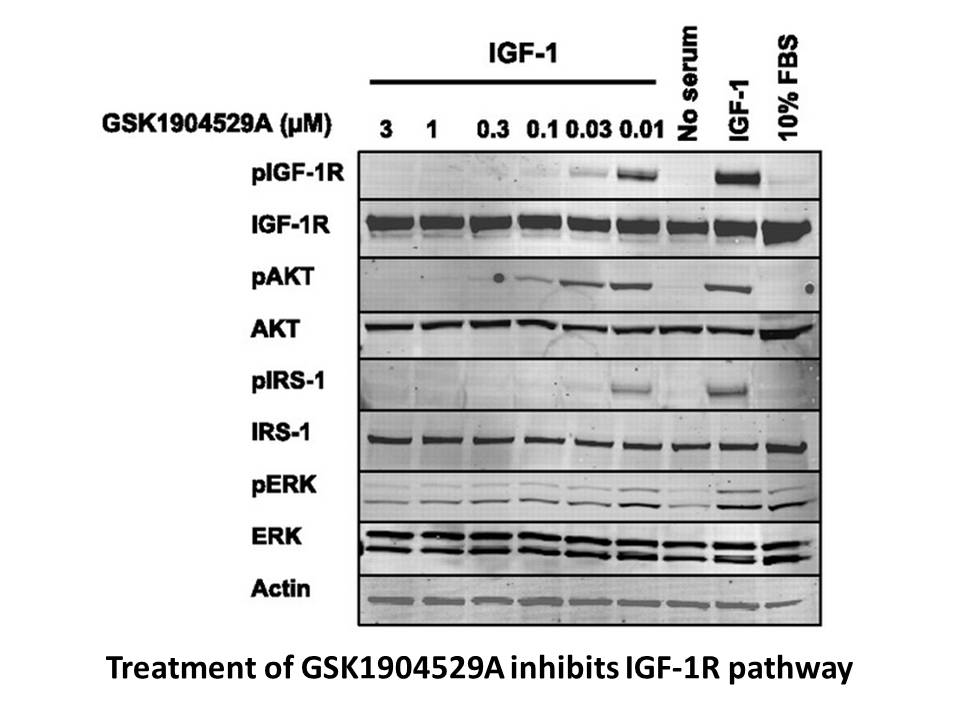### Related Biological Data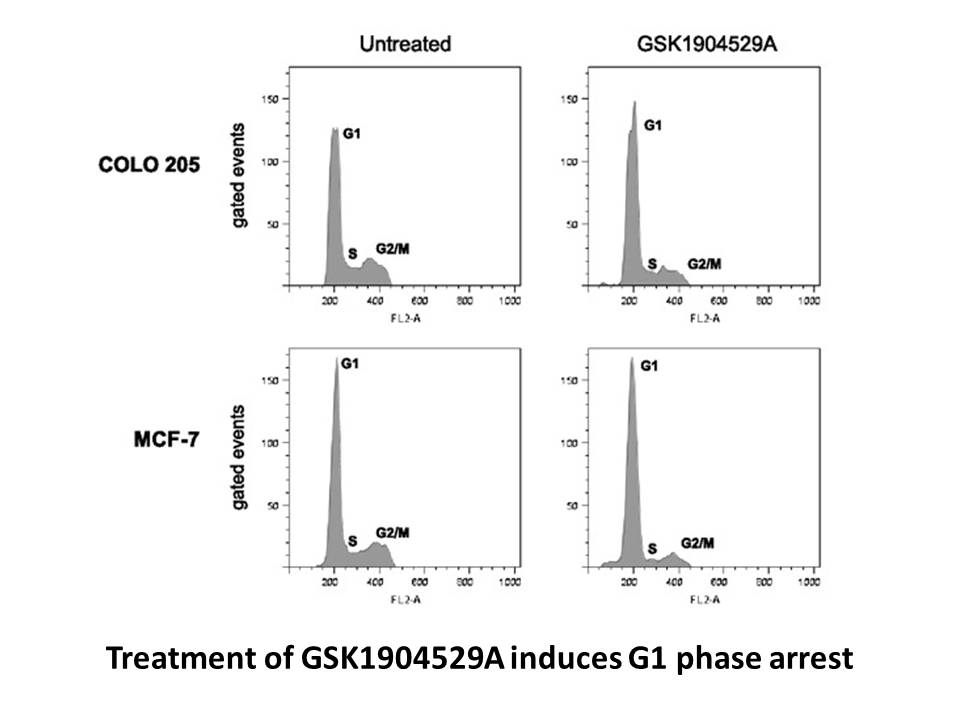### Related Biological Data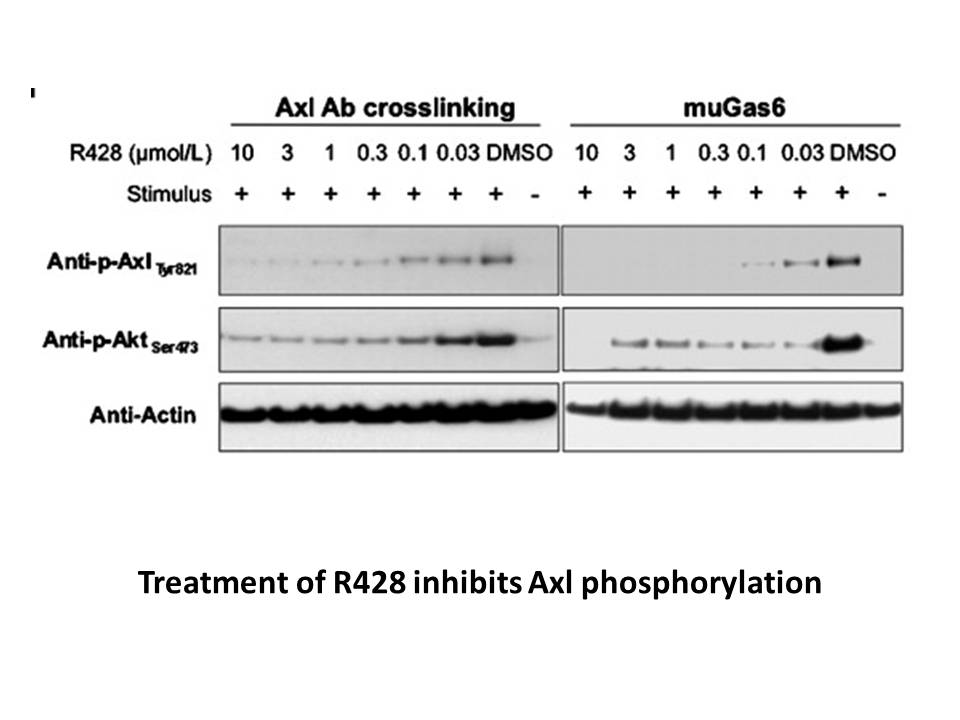### Related Biological Data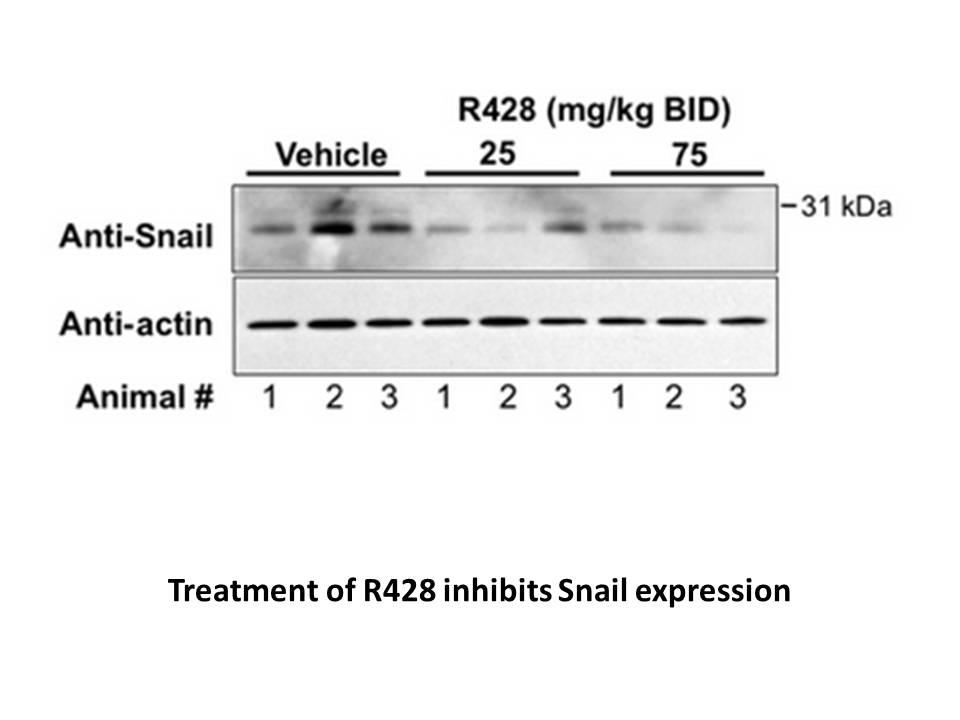### Related Biological Data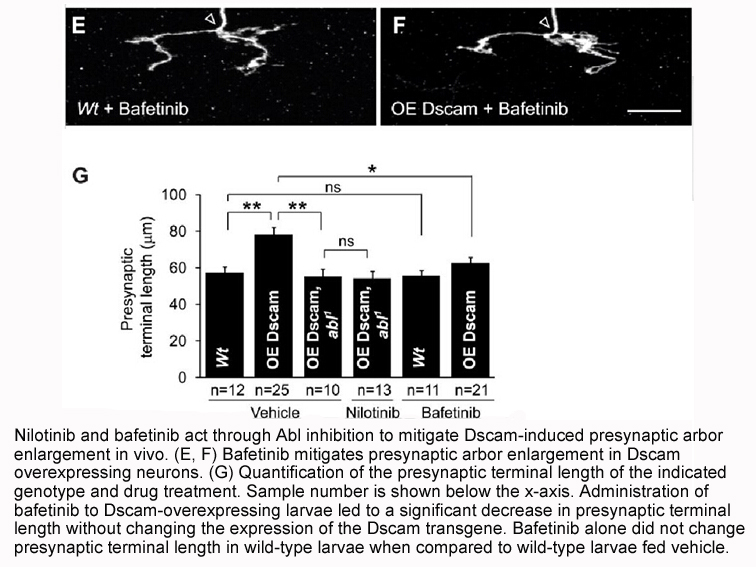## Storage and Shipping Information

 Form Pre-dissolved DMSO solutions Stability Solution: -20°C for 12 months, -80°C for 24 months Packaging 96 Well Format Sample Storage Tube With Screw Cap and Optional 2D Barcode. General tips For obtaining a higher solubility , please warm the tube at 37°C and shake it in the ultrasonic bath for a while.Stock solution can be stored below -20°C for several months. Shipping Condition Evaluation sample solution : ship with blue ice All other available size: ship with RT , or blue ice upon request

## Compound Library Composition